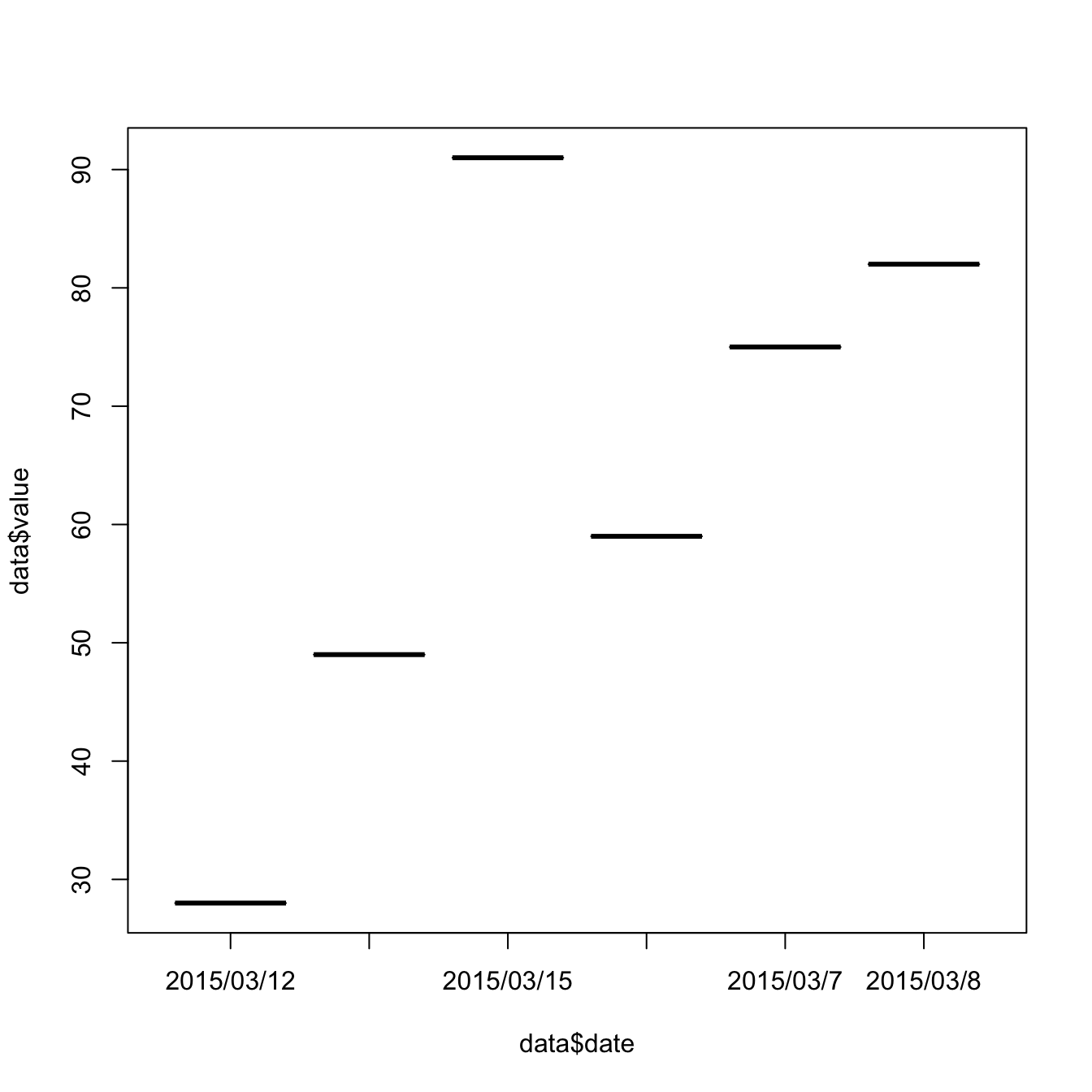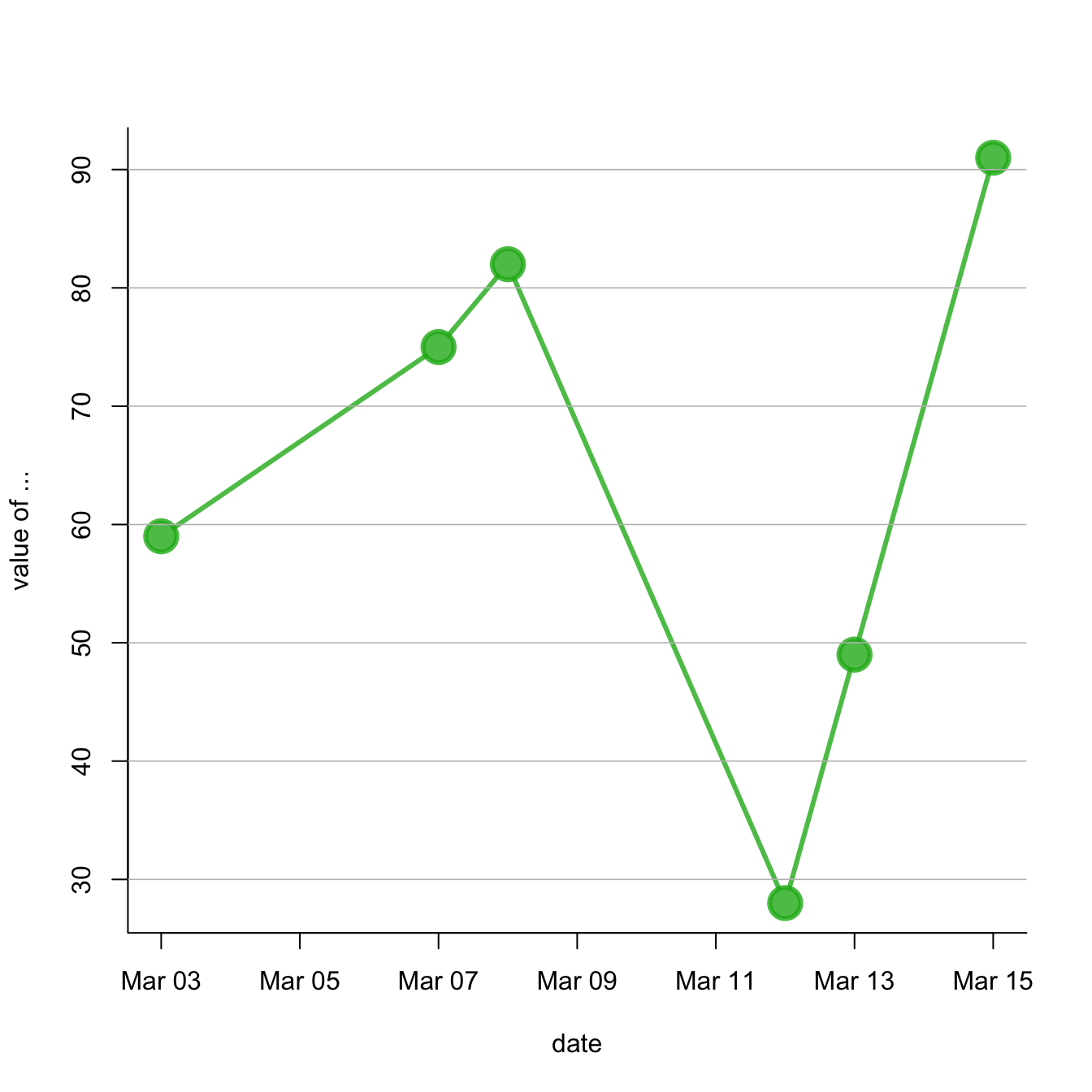# Manage dates data with base R

This post explains how to deal with date data in base R. It takes a connected scatterplot as an example and display several options to deal with dates.

# Important note about the `lubridate()` library.

I strongly advise to have a look to the `lubridate()` library. It allows to easily manipulate the date format, and is very powerfull in conjunction with ggplot2. Have a look to the time series section of the gallery.

# Is your date recognized as a date?

R offers a special data type for dates. It is important to use it since it will make the creation of charts lot easier.

The `str()` function allows to check the type of each column. In the example beside, the `date` column is recognized as a `factor`

``````# Create data
set.seed(124)
date <- paste(   "2015/03/" , sample(seq(1,31),6) , sep="")
value <- sample(seq(1,100) , 6)
data <- data.frame(date,value)

# Date and time are recognized as factor:
str(data)``````
``````## 'data.frame':    6 obs. of  2 variables:
##  \$ date : Factor w/ 6 levels "2015/03/12","2015/03/13",..: 4 2 3 1 5 6
##  \$ value: int  59 49 91 28 75 82``````

# Why it matters

The issue is that your plot is gonna be very disapointing if the date is not recognized properly, as shown beside.``````# Create data
set.seed(124)
date <- paste(   "2015/03/" , sample(seq(1,31),6) , sep="")
value <- sample(seq(1,100) , 6)
data <- data.frame(date,value)

# Date and time are recognized as factor:
#str(data)

# So ploting them works bad --> wrong order, date without value are not represented,
plot(data\$value~data\$date, type="b")``````

# Switch to date format

You can use the `as.Date()` function to specify that a column is at the date format. Now, with a bit of customization, we can get a nice connected scatterplot from our data:``````# Create data
set.seed(124)
date <- paste(   "2015/03/" , sample(seq(1,31),6) , sep="")
value <- sample(seq(1,100) , 6)
data <- data.frame(date,value)

# Let's change the date to the "date" format:
data\$date <- as.Date(data\$date)

# So we can sort the table:
data <- data[order(data\$date) , ]

# Easy to make it better now:
plot(data\$value~data\$date , type="b" , lwd=3 , col=rgb(0.1,0.7,0.1,0.8) , ylab="value of ..." , xlab="date" , bty="l" , pch=20 , cex=4)
abline(h=seq(0,100,10) , col="grey", lwd=0.8)``````

Related chart types

## Contact

This document is a work by Yan Holtz. Any feedback is highly encouraged. You can fill an issue on Github, drop me a message on Twitter, or send an email pasting yan.holtz.data with gmail.com.